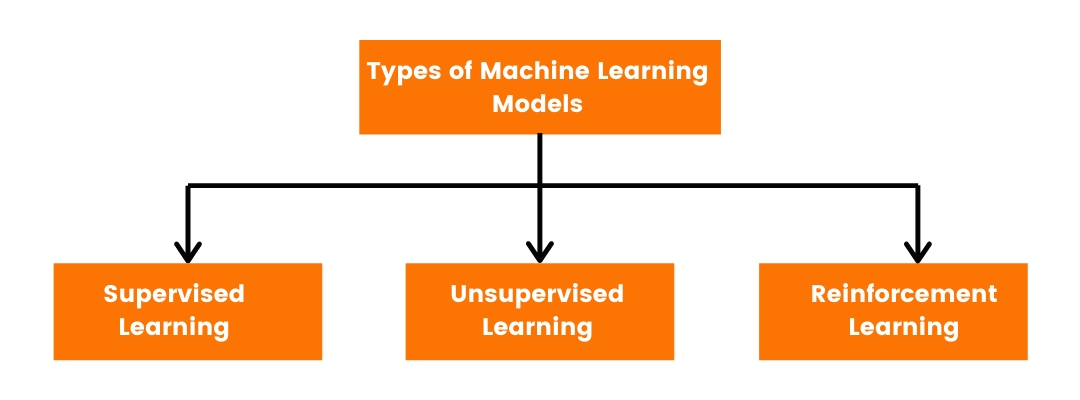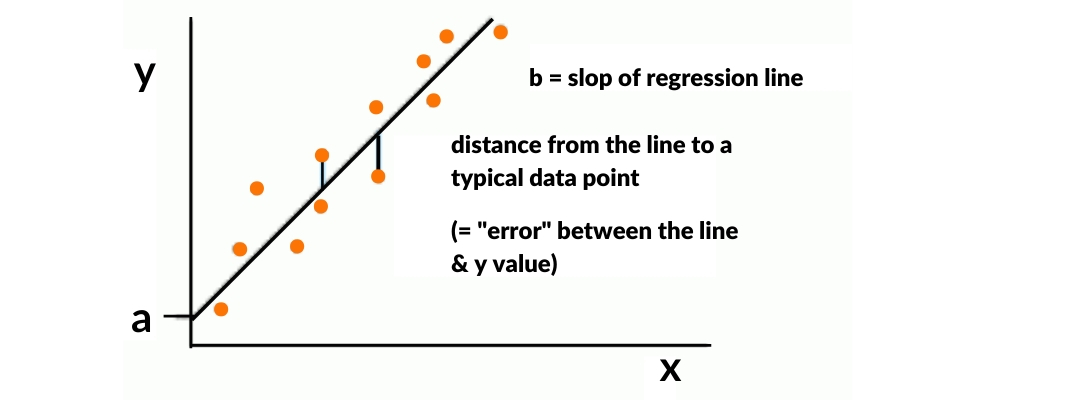# Top 10 Machine Learning Models to quick-start for Beginners

## Machine learning; An introduction

Until a couple of decades back, for most people the concept of machine learning and artificial intelligence was restricted to sci-fi movies and comic books. Now, it has encroached nearly every sphere of our lives and machines and servers are becoming increasingly reliant on machine learning models to improve their working.

Machine learning means the use of models and algorithms by a computer to augment its performance automatically by learning from experience.

In simple words, a machine learning algorithm is a logical model which analyzes patterns and employs techniques such as induction and inference to predict different outcomes and optimize the performance of the machine.

The great interest in this field is mainly because it is an emerging field and has great scope for beginners. Owing to our increased dependence on big data, machine learning is very valuable and it merges the concepts from data analyses and artificial intelligence.

Beginners usually have a great interest in this field but find themselves perplexed because there are so many machine learning algorithms to choose from and beginners can get overwhelmed.

A 2019 study shows that total funding allocated for machine learning worldwide amounts to over 28.5 Billion USD. This shows the promising future of this field.

## Types of Machine Learning ModelsWe can begin by saying that “The Perfect Machine Learning Model” simply does not exist. There are multiple models, each tailored to suit a specific purpose and each has its own pros and cons. So only after some experience, you will learn the drawbacks and advantages of individual algorithms.

For simplicity purposes, we have categorized machine learning models into three different categories:

• Supervised learning : It uses an example input-output combination and after analysis, it predicts a function that maps an input to the corresponding output. It relies on inference for computational analyses.
• Unsupervised learning : It requires little to no human supervision and itself looks for patterns in a data set. It then uses this information obtained from unknown or unlabelled outcomes, for performance enhancement.
• Reinforcement learning : It deals with how different software agents should act in order to maximize the notion of cumulative award.

## 10 Machine Learning Models to Know for Beginners

### 1. Linear Regression

It is a machine learning model based upon Supervised learning. Simply put, it explains the relationship of two variables and their mutual interdependence. It explains how change in one variable will affect the other one.

When plotted on a graph it gives a curve of the shape of y=a + bx. It is a fast and efficient machine learning model commonly used for risk assessment exercises.### 2. Logistic Regression

It can be more reasonably understood by its comparison with linear regression. In contrast to using continuous variables, it uses discrete values, which means data processing occurs in binary format.

Thus, it can be used to predict whether certain events would occur or not, whether a student would pass or fail. It extrapolates the information from a pool of data and projects the results in the form of a binary configuration.

These machine learning models/algorithms are easier to interpret and can deal with non-linear relationships as well.

Mathematically the equation for logistic regression is P(x) = e ^ (b0 +b1x) / (1 + e(b0 + b1x)).

### 3. Naive Bayes Classifier Algorithm

Let’s assume there are two independent events. Naive Bayes Machine learning model is a supervised model which calculates the odds of an event occurring, based on the information that such an event has occurred in the past while assuming no correlation between the two.

This is a beginner level machine learning model which is particularly used with massive datasets.

Mathematically, it draws upon Bayes probability theorem and uses it as a principle.

Practically, it can be used for email spam filtering, document categorization, and disease prediction models.

The accuracy of machine learning in predicting patient’s death is about 95%. This shows the prospectus future of machine learning in the healthcare industry.

### 4. Classification And Regression Tree learning

CART, also known as decision tree learning analyzes data from different sources and works its way up to the top, like branches of a tree. It is a predictive modeling approach which goes from observations about an item to the conclusion about the item’s target value.

CART models are used in data mining with the aim of creating a model that predicts the value of a target. Classification trees broadly identify the class within which the target value is likely to fall. Regression trees predict the value of a continuously variable target.

### 5. K Means Clustering Algorithm

It is a very popular “clustering” machine learning model which makes use of vector quantization. It is used to categorize unlabeled data together. It groups the data values together in different clusters and assigns each a value. Then the algorithm makes predictions for example about the chances that a particular customer would return to the shop or not.

It has been found that 80% of companies plan to adopt artificial intelligence to gauge customer service.

It is also used by many search engines like Bing and Yahoo to cluster different “related results” together.

### 6. PCA

Next on the list is Principal Component Analysis which is also an unsupervised machine learning model. It is used for dimensionality reduction and uses an orthogonal transformation to convert a group of correlated variables to a set of uncorrelated variables. This statistical technique makes data easy to visualize and explore, by reducing the number of variables.

### 7. SVM (Support Vector Machine)

This is a supervised machine learning algorithm which works best with relatively smaller datasets but at the same time, its ability to interpret complex patterns makes it a desirable model to begin with. It relies on a kernel trick to transform the data and then uses these transformations to find an optimal boundary between different outputs.

### 8. Artificial Neural Networks

Human mind and the billions of neural connections have always intrigued scientists. ANN takes its inspiration from the neuronal computational ability of the human mind. It has two layers; namely input and output layers. ANN are used to detect patterns in datasets which are either too big or too complex for humans to understand and feed into a machine.

These machine learning algorithms are typically used for ensemble formation. It is a gradient-descent based formulation of the boosting method. They are highly flexible and this makes them desirable for particular data-driven tasks. They have found great application in neurorobotics where they can be used to perform the predictive function.

### 10. Random Forest

Machine learning model would be incomplete without mentioning Random Forest Algorithms. It is a widely used model that can produce accurate results even without any type of hyper-parameter tuning. Basically, it is an ensemble of decision trees, usually trained with a bagging method. This uses multiple learning models and merges their findings to produce optimum results.

Machine learning is an emerging field which means that there is a lot of room for personal growth and exploration. The drawback would be that you get to learn a lot of things, only after having your share of trial-and-error. Make sure you get a solid foundation by working your way through any one of the suggested beginner-level machine learning languages, and it would be a smooth ride from there.

Looking for a job on Machine learning? We can help! Click Here

The Job Fair for Tech & Digital Professionals, A great opportunity to find or change to a new job, learn from the best experts in the IT & Digital industry, and network with your peers. Get Your Free Ticket

# Join 20,000+ Subscribers